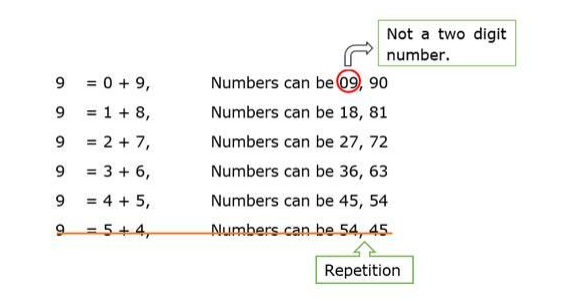# Write the following sets in roster from:

Question:

Write the following sets in roster from:

C = {x : x is a two-digit number such that the sum of its digits is 9}.

Solution:The elements of this set are 18, 27, 36, 45, 54, 63, 72, 81 and 90

So, C = {18, 27, 36, 45, 54, 63, 72, 81, 90}